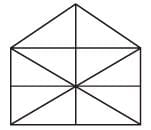Olympiad Test: Analytical Reasoning - 1

# Olympiad Test: Analytical Reasoning - 1 - Class 8

Test Description

## 10 Questions MCQ Test Mathematical Olympiad Class 8 - Olympiad Test: Analytical Reasoning - 1

Olympiad Test: Analytical Reasoning - 1 for Class 8 2023 is part of Mathematical Olympiad Class 8 preparation. The Olympiad Test: Analytical Reasoning - 1 questions and answers have been prepared according to the Class 8 exam syllabus.The Olympiad Test: Analytical Reasoning - 1 MCQs are made for Class 8 2023 Exam. Find important definitions, questions, notes, meanings, examples, exercises, MCQs and online tests for Olympiad Test: Analytical Reasoning - 1 below.
 1 Crore+ students have signed up on EduRev. Have you?
Olympiad Test: Analytical Reasoning - 1 - Question 1

### Direction: In each of the following problems, find the number of triangles in the given figure: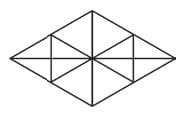Detailed Solution for Olympiad Test: Analytical Reasoning - 1 - Question 1

12 + 8 + 4 + 4 = 28

Olympiad Test: Analytical Reasoning - 1 - Question 2

### Direction: In each of the following problems, find the number of triangles in the given figure: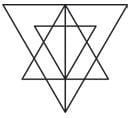Detailed Solution for Olympiad Test: Analytical Reasoning - 1 - Question 2

8 + 10 + 5 + 2 + 1 + 1 = 27.

Olympiad Test: Analytical Reasoning - 1 - Question 3

### Direction: In each of the following problems, find the number of triangles in the given figure: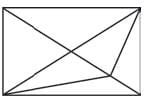Detailed Solution for Olympiad Test: Analytical Reasoning - 1 - Question 3

6 + 6 + 2 + 1 + 15

Olympiad Test: Analytical Reasoning - 1 - Question 4

Direction: In each of the following problems, find the number of triangles in the given figure: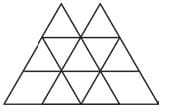Detailed Solution for Olympiad Test: Analytical Reasoning - 1 - Question 4

10 + 2 + 4 + 2 = 18

Olympiad Test: Analytical Reasoning - 1 - Question 5

Direction: In each of the following problems, find the number of triangles in the given figure: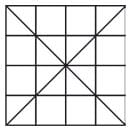Detailed Solution for Olympiad Test: Analytical Reasoning - 1 - Question 5

16 + 4 + 8 + 4 + 4 + 8 + 4 = 48

Olympiad Test: Analytical Reasoning - 1 - Question 6

Direction: In each of the following problems, find the number of triangles in the given figure: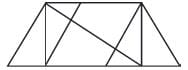Detailed Solution for Olympiad Test: Analytical Reasoning - 1 - Question 6

6 + 4 + 3 + 1 = 14

Olympiad Test: Analytical Reasoning - 1 - Question 7

Direction: In each of the following problems, find the number of triangles in the given figure: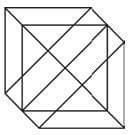Olympiad Test: Analytical Reasoning - 1 - Question 8

Direction: In each of the following problems, find the number of triangles in the given figure: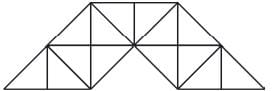Olympiad Test: Analytical Reasoning - 1 - Question 9

Direction: In each of the following problems, find the number of triangles in the given figure: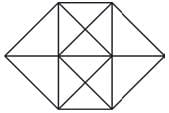Olympiad Test: Analytical Reasoning - 1 - Question 10

Direction: In each of the following problems, find the number of triangles in the given figure: